# Motion in One Dimension Chapter Exam

Exam Instructions:

Choose your answers to the questions and click 'Next' to see the next set of questions. You can skip questions if you would like and come back to them later with the yellow "Go To First Skipped Question" button. When you have completed the practice exam, a green submit button will appear. Click it to see your results. Good luck!

### Page 5

#### Question 21 21. Two drones are flying in the x-z plane. What is the proper vector-addition setup to solve for the velocity of drone 2 relative to drone 1? Drone 1 is represented by the number 1 and drone 2 is represented by the number 2.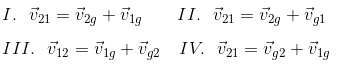#### Question 22 22. Which of the displacements is two-dimensional?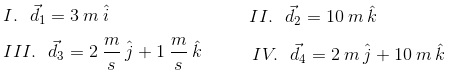#### Question 23 23. An airplane and a drone are flying at the given velocities which are both relative to the air. What is the velocity of the airplane relative to the drone in the z-direction?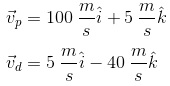#### Question 24 24. The cue ball and the eight ball are moving relative to each other on a pool table. How fast is the eight ball moving in the y-direction relative to the pool table? 'e' stands for eight ball, 'c' stands for cue ball, and 't' stands for table.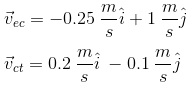#### Question 25 25. The data below gives the velocities and partial acceleration of a 6 kg mass. What is the acceleration of the object in the y-direction?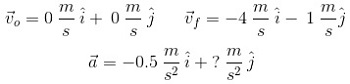### Page 6

#### Question 26 26. Calculate the average speed for the course, if you walked the black line in 1.7 hours.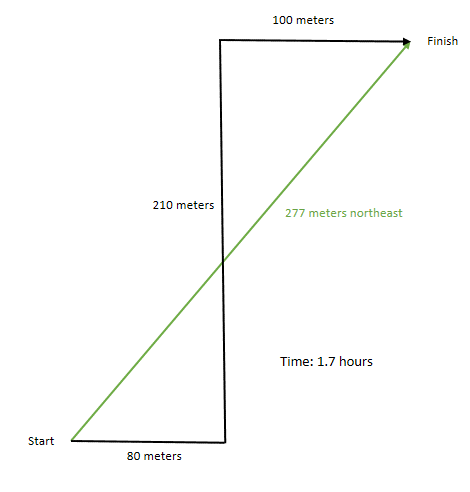#### Question 28 28. Calculate the average velocity for the course, if you walked the black line in 1.7 hours.#### Motion in One Dimension Chapter Exam Instructions

Choose your answers to the questions and click 'Next' to see the next set of questions. You can skip questions if you would like and come back to them later with the yellow "Go To First Skipped Question" button. When you have completed the practice exam, a green submit button will appear. Click it to see your results. Good luck!

Support